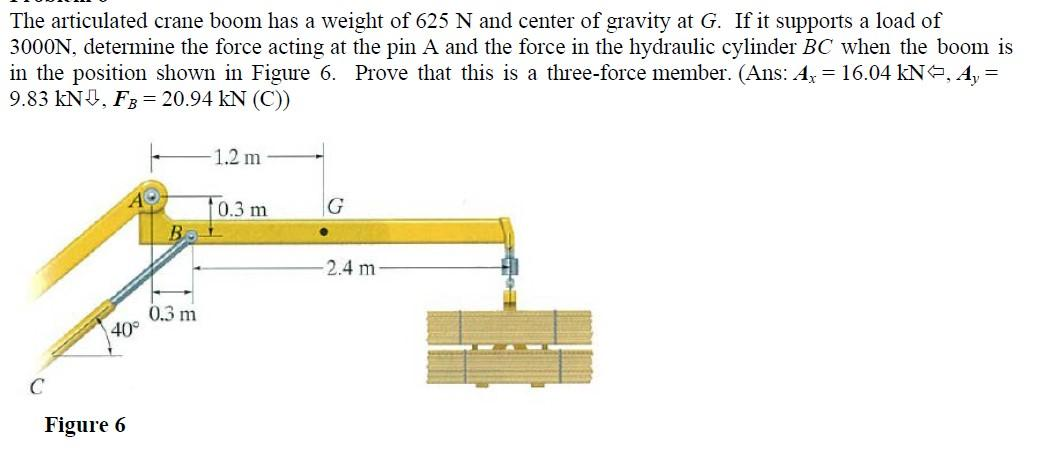# Question The articulated crane boom has a weight of 625 N and center of gravity at G. If it supports a load of 3000N, determine the force acting at the pin A and the force in the hydraulic cylinder BC when the boom is in the position shown in Figure 6. Prove that this is a three-force member. (Ans: Az = 16.04 kN, A, = 9.83 kND, FB = 20.94 kN (C) 1.2 m 10.3 m G 2.4 m 0.3 m 40° С Figure 6YZT0YX The Asker · Mechanical EngineeringTranscribed Image Text: The articulated crane boom has a weight of 625 N and center of gravity at G. If it supports a load of 3000N, determine the force acting at the pin A and the force in the hydraulic cylinder BC when the boom is in the position shown in Figure 6. Prove that this is a three-force member. (Ans: Az = 16.04 kN, A, = 9.83 kND, FB = 20.94 kN (C) 1.2 m 10.3 m G 2.4 m 0.3 m 40° С Figure 6
More
Transcribed Image Text: The articulated crane boom has a weight of 625 N and center of gravity at G. If it supports a load of 3000N, determine the force acting at the pin A and the force in the hydraulic cylinder BC when the boom is in the position shown in Figure 6. Prove that this is a three-force member. (Ans: Az = 16.04 kN, A, = 9.83 kND, FB = 20.94 kN (C) 1.2 m 10.3 m G 2.4 m 0.3 m 40° С Figure 6